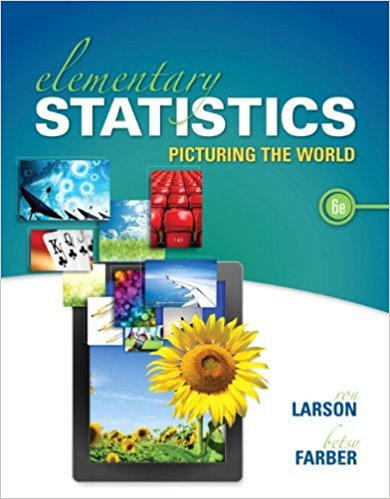×
×

# Solutions for Chapter 9.2: CORRELATION AND REGRESSION## Full solutions for Elementary Statistics: Picturing the World | 6th Edition

ISBN: 9780321911216Solutions for Chapter 9.2: CORRELATION AND REGRESSION

Solutions for Chapter 9.2
4 5 0 342 Reviews
13
2
##### ISBN: 9780321911216

Since 48 problems in chapter 9.2: CORRELATION AND REGRESSION have been answered, more than 109199 students have viewed full step-by-step solutions from this chapter. This expansive textbook survival guide covers the following chapters and their solutions. Elementary Statistics: Picturing the World was written by and is associated to the ISBN: 9780321911216. This textbook survival guide was created for the textbook: Elementary Statistics: Picturing the World , edition: 6. Chapter 9.2: CORRELATION AND REGRESSION includes 48 full step-by-step solutions.

Key Statistics Terms and definitions covered in this textbook
• Acceptance region

In hypothesis testing, a region in the sample space of the test statistic such that if the test statistic falls within it, the null hypothesis cannot be rejected. This terminology is used because rejection of H0 is always a strong conclusion and acceptance of H0 is generally a weak conclusion

A formula used to determine the probability of the union of two (or more) events from the probabilities of the events and their intersection(s).

• Assignable cause

The portion of the variability in a set of observations that can be traced to speciic causes, such as operators, materials, or equipment. Also called a special cause.

• Average

See Arithmetic mean.

• Central composite design (CCD)

A second-order response surface design in k variables consisting of a two-level factorial, 2k axial runs, and one or more center points. The two-level factorial portion of a CCD can be a fractional factorial design when k is large. The CCD is the most widely used design for itting a second-order model.

• Comparative experiment

An experiment in which the treatments (experimental conditions) that are to be studied are included in the experiment. The data from the experiment are used to evaluate the treatments.

• Conditional variance.

The variance of the conditional probability distribution of a random variable.

• Confounding

When a factorial experiment is run in blocks and the blocks are too small to contain a complete replicate of the experiment, one can run a fraction of the replicate in each block, but this results in losing information on some effects. These effects are linked with or confounded with the blocks. In general, when two factors are varied such that their individual effects cannot be determined separately, their effects are said to be confounded.

• Continuity correction.

A correction factor used to improve the approximation to binomial probabilities from a normal distribution.

• Continuous distribution

A probability distribution for a continuous random variable.

• Continuous uniform random variable

A continuous random variable with range of a inite interval and a constant probability density function.

• Correlation matrix

A square matrix that contains the correlations among a set of random variables, say, XX X 1 2 k , ,…, . The main diagonal elements of the matrix are unity and the off-diagonal elements rij are the correlations between Xi and Xj .

• Crossed factors

Another name for factors that are arranged in a factorial experiment.

• Error of estimation

The difference between an estimated value and the true value.

• Error variance

The variance of an error term or component in a model.

• Experiment

A series of tests in which changes are made to the system under study

• Factorial experiment

A type of experimental design in which every level of one factor is tested in combination with every level of another factor. In general, in a factorial experiment, all possible combinations of factor levels are tested.

• Finite population correction factor

A term in the formula for the variance of a hypergeometric random variable.

• Frequency distribution

An arrangement of the frequencies of observations in a sample or population according to the values that the observations take on

• Harmonic mean

The harmonic mean of a set of data values is the reciprocal of the arithmetic mean of the reciprocals of the data values; that is, h n x i n i = ? ? ? ? ? = ? ? 1 1 1 1 g .

×